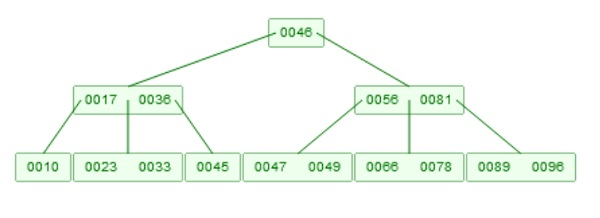# B-tree Query in Data Structure

Here we will see, how to perform the searching in B-Tree. The B-Tree searching is also known as B-Tree Querying. Suppose we have a B-tree like below −

Example of B-TreeThe searching technique is very similar to the binary search tree. Suppose we want to search 66 from the above tree. So we will start from root, now 66 is larger than root element 46. So we will move to the right child of the root. Then the right child has more than one element. The elements are sorted, they are [56, 81]. Our target key is larger than 56, but smaller than 81, so we will enter into the subtree, which is in between 56 and 81. So then we have moved to the leaf level. At that point we have got the element 66.

Let us see the algorithm to search element inside the B-Tree.

## Algorithm

BTreeSearch(root, key)

Input − The root of the tree, and key to find

Output − The value of the node with key, if it is not present, return null

x := Read root
if x is an index node, then
if there is an object o in x, such that o->key = ‘key’, then return o->val
Find the child of x, as x->child[i], whose key range is containing ‘key’
return BTreeSearch(x->child[i], key)
else
if there is an object o in x, such that o->key = ‘key’, then return o->val,
else return null
end if
end if

Updated on: 11-Aug-2020

355 Views